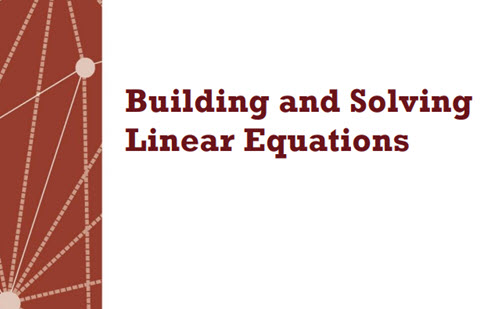Go to website

# Building linear equations

This sequence of lessons explores how well students can create and solve linear equations. In particular the tasks identify students who are able to solve equations with one variable and can solve equations in more than one way. Students work on a task independently, then work collaboratively in pairs, and finally with the whole class. Full lesson plans and slides are provided for the teacher. Guidance is given on how to interpret students' responses to diagnose misconceptions and provide follow-up lessons.

Year level(s) Year 8
Audience Teacher
Teaching strategies and pedagogical approaches Feedback
Keywords Linear, Equations, Variable

## Curriculum alignment

Curriculum connections Numeracy
Strand and focus Algebra
Topics Linear relationships, Algebraic expressions
AC: Mathematics (V9.0) content descriptions
AC9M8A01
Create, expand, factorise, rearrange and simplify linear expressions, applying the associative, commutative, identity, distributive and inverse properties

Numeracy progression Number patterns and algebraic thinking (P7)